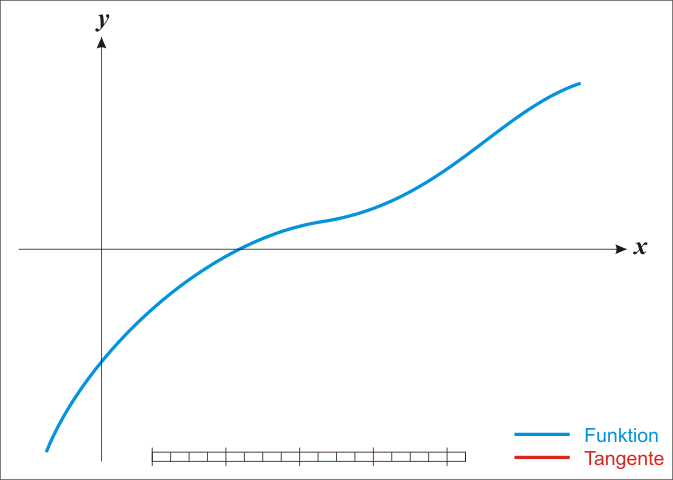# Computing the Square Root with Newton's Method

Written by Toni Karppi on

In this article we’ll be looking at an algorithm that can be used to solve square roots: Newton’s method. Newton’s method is a root-finding algorithm which takes advantage of calculus and algebra to find its solutions. The algorithm works iteratively, where we start with an initial guess for the root, and then we use information from tangent lines to find better and better approximations of the roots. Here’s an animation by Ralf Pfeifer that demonstrates this process.## Derivation of Newton’s Method

We have a non-linear function $f(x)$, and we would like to find it’s roots, i.e. the points where it touches the x-axis, $f(x) = 0$. In general, finding the roots of $f(x)$ is non-trivial. On the other hand, finding the intersection of a straight line and the x-axis is easy. So the trick here is to first guess a value for the root; then from that point we calculate the tangent line, and see where it crosses the x-axis. The equation for the tangent line at point $k$ is given by:

$y = f'(k)(x-k)+f(k)$

To find the intersections with the x-axis, we set $y = 0$. Using simple algebraic manipulation, we can rewrite the equation as:

$x = k-{f(k) \over f'(k)}$

So the tangent crosses the x-axis when $x = k-f(k)/f'(k)$. We can now use this value as a new guess, and repeat this proess. In most cases this algorithm converges to a root.

We can rewrite the above formula as:

$x_{i+1} = x_i-{f(x_i) \over f'(x_i)}$

Let’s look at an example of Newton’s method. In this case we want to compute the value of $\sqrt{2}$. We can write this as $x^2 = 2$, where $x$ is the solution. We can rewrite this as $x^2 - 2 = 0$, so the solutions are given by the roots of the function $f(x) = x^2 - 2$. Next we compute the derivative: $f'(x) = 2x$. Since $\sqrt{x} <= x$, a decent intial guess for the root is $x/2$. Thus, for $x = 2$, we would choose $x = 1$ as our intial guess.

## Programming a square root function

Now let’s program a square root function to see this in practice.

def square_root(n):
x = n / 2
for i in range(100):
fx = x * x - n
fpx = 2 * x
x = x - fx / fpx

return x

result = square_root(2)
print(result)

Here we run for 100 iterations, and then print out the result. This program prints out the solution $1.414213562373095$. Now running for a constant number of iterations may not be what we want, since we don’t really get a good sense whether the result is close to a root, and we may also be performing unnecessary iterations. A better way is to check if we have converged to a point. This can be done by checking the distance from the current point to the previous point.

def square_root(n):
x = n / 2
x_prev = None
while x_prev is None or abs(x_prev - x) > 1e-9:
x_prev = x
fx = x * x - n
fpx = 2 * x
x = x - fx / fpx

return x

result = square_root(2)
print(result)

Currently the program contains some bugs. You can try running the program with $n = 0$, or $n < 0$. In the first case you should see a ZeroDivisionError, and in the second case you get stuck in an infite loop. We can patch these two cases with if-checks.

def square_root(n):
if n == 0:
return 0
if n < 0:
raise ValueError("The input value must be non-negative.")

x = n / 2
x_prev = None
while x_prev is None or abs(x_prev - x) > 1e-9:
x_prev = x
fx = x * x - n
fpx = 2 * x
x = x - fx / fpx

return x

result = square_root(2)
print(result)

We can actually simplify the program a bit using basic algebra.

$x - {x^2 - n \over 2x} = 0 \iff {x + {n \over x} \over 2} = 0$
def square_root(n):
if n == 0:
return 0
if n < 0:
raise ValueError("The input value must be non-negative.")

x = n / 2
x_prev = None
while x_prev is None or abs(x_prev - x) > 1e-9:
x_prev = x
x = (x + n / x) / 2

return x

result = square_root(2)
print(result)

There we go; we have now implemented the square root function using Newton’s method. Remember that Newton’s method can also be used for other functions that you want to find roots for. There’s one thing however that you should keep in mind if you’re going to use Newton’s Method: It does not guartantee convergence. You may need to find a fallback algorithm if Newton’s method doesn’t converge within a fixed number of iterations. One example of a case where no progress would be made is if the derivative evaluated to $0$, since then there is no intersection with the x-axis. The algorithm for the square root given above does converge for all allowed values, but that’s a special case resulting from the shape of the function.